BBC BASIC « Using SIN, RAD, COS to draw angles and a circle » Welcome Guest. Please Login or Register.Mar 31st, 2018, 10:43pm

ATTENTION MEMBERS: Conforums will be closing it doors and discontinuing its service on April 15, 2018.
We apologize Conforums does not have any export functions to migrate data.
Ad-Free has been deactivated. Outstanding Ad-Free credits will be reimbursed to respective payment methods.

Thank you Conforums members.
 Cross-platform BBC BASIC (Windows, Linux x86, Mac OS-X, Android, iOS, Raspberry Pi) BBC BASIC Resources BBC BASIC Help Documentation BBC BASIC for Windows Home Page BBC BASIC Programmers' Reference BBC BASIC Beginners' Tutorial BBC BASIC for SDL 2.0 Home Page BBC BASIC Discussion Group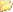BBC BASIC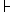Programming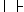BBC BASIC Language (Moderator: Richard Russell)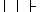Using SIN, RAD, COS to draw angles and a circle « Previous Topic | Next Topic »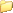Author Topic: Using SIN, RAD, COS to draw angles and a circle  (Read 141 times)
michael
Full Member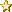member is offline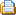Posts: 157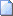Using SIN, RAD, COS to draw angles and a circle « Thread started on: Nov 8th, 2017, 02:28am »

This example appears to be 3 times faster on BBCSDL..

It would be interesting to know how to create depth also with this method.

Uses: could be used for clock displays, gauges, and other indicators.
FNhdegdist(degrees,length)-for returning a horizontal location
FNvdegdist(degrees,length)-for returning a vertical location

Of course you would combine them into a line or many lines:
LINE 300,300,300+FNhdegdist(45,100),300+FNvdegdist(45,100)
**** creates a line at 300,300 with a length of 300 pixels at a angle of 45 degrees.

and then there is:

PROC_anglelocationdist(degrees%,sx%,sy%,RETURN vs%,RETURN vy%)
sx% and sy% really just hold the length (100) --I could have made one number, but to show how it relates to horizontal and vertical lengths I did it this way.

example:
PROC_anglelocationdist(90,100,100,h%,v%)
would return a 90 degree 100 pixel lengths coordinates for h% and v%
And h% and v% would be replaced with variables you are using.

If you want you could change this procedure to:

DEF PROC_anglelocationdist(degrees%,dist%,RETURN vs%,RETURN vy%)

and then you would discard sx% and sy% and replace them with dist%
Code:
```      MODE 8
REM if you are reading this, you are seeing an experiment in progress..  unknown effects (ok its known)

GCOL 3
PROC_myellipse(200,200,100,150):GCOL 2:PROC_myellipse(200,500,150,100)
REM draw a line from 300,300 to 100 points in a 45 degree angle
LINE 300,300,300+FNhdegdist(45,100),300+FNvdegdist(45,100)
VDU 23,23,3|
dv%=0:i=0
FOR dv%=1 TO 150 STEP 2
i+=2.5
COLOUR 0,250-i,200-i,210-i : GCOL 0
PROC_myellipse(800,500,0+dv%,0+dv%)
NEXT dv%
END
REM horizontal degrees and length
DEF FNhdegdist(degrees%,sx%)
=vs%
REM vertical degrees and length
DEF FNvdegdist(degrees%,sy%)
=vy%
REM using this Procedure, sx%*SIN RAD gives the Horizontal distance in radians (sx%-requested distance)
DEF PROC_anglelocationdist(degrees%,sx%,sy%,RETURN vs%,RETURN vy%)
ENDPROC
REM x,y sizex, sizey
DEF PROC_myellipse(x,y,sx,sy)
LOCAL xs%,yc%,d%
FOR d%=0 TO 360
PROC_anglelocationdist(d%,sx,sy,xs%,yc%):REM 45 degree angle at a scale of 100
LINE x+xs%,y+yc%,x+xs%,y+yc%
NEXT d%
ENDPROC
REM PROC_tri(x,y,size,R,G,B,dimmer)
DEFPROC_tri(x,y,s,r%,g%,b%,d_i%)
REM center will be x,y
LOCAL x%,skip%
skip%=FALSE
FOR x%=0 TO s
r%=r%-d_i%
g%=g%-d_i%
b%=b%-d_i%
IF r% <2 THEN r%=2
IF g% <2 THEN g%=2
IF b%<2 THEN b%=2
IF r%<50 AND g%<50 AND b%<50 THEN skip%=TRUE
IF skip%=FALSE THEN
COLOUR 1,r%,g%,b%:GCOL 1
PROC_triangle(x,y,x%)
ENDIF
NEXT x%
MOVE 0,0
ENDPROC
REM PROC_triangle(x,y,size)- Just a sizable triangle. you set color
DEFPROC_triangle(x,y,s%)
LOCAL c%
c%=s%/2
m%=s%/4
LINE x-c%,y,x,y-m%
LINE x,y-m%,x+c%,y
REM LINE x-c%,y,x+c%,y
LINE x-1+c%,y+1,x,y+s%
LINE x,y+s%,x-c%+1,y+1
ENDPROC
```
 « Last Edit: Nov 8th, 2017, 03:38am by michael »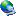Logged

I like reinventing the wheel, but for now I will work on tools for D3D
 Forum Jump ------------------ => General ------------------ - General Board - Announcements - Support and Promote ------------------ => Development ------------------ - Interpreter & Run-Time Engine - Integrated Development Environment - Linux (86) - Mac OS-X - Android - Raspberry Pi - iOS ------------------ => Programming ------------------ - BBC BASIC Language - Operating System Interface - VDU Emulation - Libraries - Assembly Language - Communication and Input/Output - Database and Files - Graphics and Games - Sound, Music and Video - User Interface « Previous Topic | Next Topic »

| |